# Correlation of data: linear regression

Linear regression is used to see whether one continuous variable is correlated with another continuous variable in a linear way, i.e. can the dependent variable y be modelled with a straight-line response to changes in the independent covariate x:$y = a + bx + \epsilon$

Here b is the estimated slope of the best-fit line (a.k.a. gradient, often written m), a is its y-intercept (often written c), and ϵ is the residual error. If the x and y data are perfectly correlated, then ϵ=0 for each and every x,y pair in the in the data set; however, this is extremely unlikely to occur in real-world data.

When you fit a linear model like this to a data set, each coefficient you fit (here, the intercept and the slope) will be associated with a t value and p value, which are essentially the result of a one-sample t test comparing the fitted value to 0.

Linear regression is only valid if:

• The x and y variables have a linear relationship. If y is a function of the square of x, or has a hyperbolic relationship with x, then naïve linear regression must not be used, as it will try to fit a straight line to what is clearly not a straight-line relationship. It is often possible to transform curved relationships to straight-line relationships using transformation (logs, reciprocals, etc.) Always plot() and eyeball your data before modelling! A salutary warning of what happens when you don’t plot your data first is Anscombe’s Quartet.
• The data sets are representative of the larger population. As usual, if you collect data that is biased, fraudulent or of very small size, then any kind of statistical analysis is likely to be broken.
• The residuals are normally distributed and homoscedastic. When the linear model is fitted, there will be some residual ‘noise’, i.e. the ϵ error term above. These residuals must be normally distributed, and should not be a function of the value of the x variable, i.e. the variance of y data at small values of x should be the same as the variance of y data at large values of x.
• Each pair of data is independent. Each x,y pair should be independent of every other x,y pair.
• The x variable is measured without error. Only the y variable can have an error associated with it.

Linear regression is very commonly used in circumstances where it is not technically appropriate, e.g. time-series data (where later x,y pairs are most certainly not independent of earlier pairs), or where the x-variable does have some error associated with it (e.g. from pipetting errors), or where a transformation has been used that will make the residuals non-normal. You should at least be aware you are breaking the assumptions of the linear regression procedure if you use it for data of this sort.

The file cricket_chirps.csv contains data on the frequency of cricket chirps (Hz) at different temperatures (°C). A quick plot of the data seems to show a positive, linear relationship:

cricket.chirps<-read.csv( "H:/R/cricket_chirps.csv" )
plot(
Frequency ~ Temperature,
data = cricket.chirps,
xlab = "Temperature / °C",
ylab = "Frequency / Hz",
main ="Crickets chirp more frequently at higher temperatures",
pch  = 15
# The pch option can be used to control the pointer character
)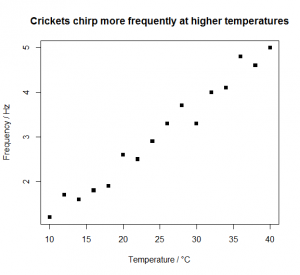To model the data, you need to use lm( y ~ x, data=data.frame ). The lm() stands for “linear model”.

lm( Frequency ~ Temperature, data=cricket.chirps )
Call:
lm(formula = Frequency ~ Temperature, data = cricket.chirps)
Coefficients:
(Intercept)       Temperature
-0.1140       0.1271

You’ll often want to save the model for later use, so you can assign it to a variable. summary() can then be used to see what R thinks the slope and intercept are:

chirps.model<-lm( Frequency ~ Temperature, data=cricket.chirps )
summary( chirps.model )
Call:
lm(formula = Frequency ~ Temperature, data=cricket.chirps)

Residuals:
Min       1Q    Median       3Q       Max
-0.39779 -0.11544  -0.00191  0.12603   0.33985

Coefficients:

Estimate Std. Error t value Pr(>|t|)
(Intercept) -0.113971   0.152264  -0.749    0.467
Temperature  0.127059   0.005714  22.235 2.55e-12 ***
---
Signif. codes:
0 '***' 0.001 '**' 0.01 '*' 0.05 '.' 0.1 ' ' 1
Residual standard error: 0.2107 on 14 degrees of freedom
Multiple R-squared:
F-statistic: 494.4 on 1 and 14 DF,  p-value: 2.546e-12
• The (Intercept) is a from the formula at the top of this section. It is the y-intercept of the line of best fit through the x,y data pairs. It is value of y (Frequency) when x (Temperature) is zero, i.e. how frequently the crickets chirp at the freezing point of water. The estimated value is -0.1140 Hz, which is impossible(!), but satisfyingly does not appear to be significantly different from zero (p=0.467).
• The Temperature is b from the formula at the top of this section. It is the slope of line of best fit through the x,y data pairs. The estimated value is 0.1271 Hz °C−1, i.e. for every 10°C increase in temperature, the chirping rate increases by about 1.3 Hz.
• The Multiple R-squared value is the square of the correlation coefficient R for Frequency on Temperature. Values of R2 close to 1 indicate y is well correlated with the x covariate with relatively little scatter; values close to 0 indicate the scatter is large and x is a poor predictor of y.

To understand the meaning of the F-statistic part of the report, it is important to understand what actually happens when you perform a linear regression. What you’re really trying to find out in linear regression is whether a straight-line with non-zero slope “y=a+bx” is a better model of the dependent variable than a straight line of slope zero, with a y-intercept equal to a constant, usually the mean of the y values: “y=“. These two possible models are shown below. The code needed to display them with the deviance of each datum from the regression line picked out as red (col="red") vertical line segments is also shown.

Linear regression model “y=a+bx” with non-zero slope…

chirps.model<-lm( Frequency ~ Temperature, data=cricket.chirps )
abline( chirps.model )
chirps.predicted<-data.frame( Temperature=cricket.chirps$Temperature ) chirps.predicted$Frequency<-predict( chirps.model, newdata=chirps.predicted )
segments(
cricket.chirps$Temperature, cricket.chirps$Frequency,
chirps.predicted$Temperature, chirps.predicted$Frequency,
col="red"
)

We use the predict() function to predict the Frequency values from the model and a data frame containing Temperature values. We put the predicted Frequency values into a new column in the data frame by assigning them using the $ dollar syntax. To add a regression line to the current plot using the fitted model, we use abline(). Like many of the other functions we’ve seen, this can either take an explicit intercept and slope: # abline( a=intercept, b=slope ) abline( a=-0.1140, b=0.1271 ) Or it can take a tilde ~ modelled-by formula: abline( cricket.chirps.model ) We use the segments() function to add red line segments to represent the deviation of each datum from the regression line. segments() takes four vectors as arguments, the x and y coordinates to start each segment from (here, the measured Temperature, Frequency data points), plus the x and y coordinates to finish each line (the equivalent columns from the data frame containing the predicted data: these are the corresponding points on the regression line).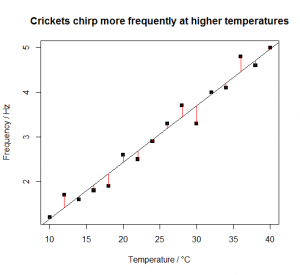y is a constant “y= ” model with zero slope… mean.frequency<-mean( cricket.chirps$Frequency )
abline( a=mean.frequency, b=0 )
segments(
cricket.chirps$Temperature, cricket.chirps$Frequency,
cricket.chirps$Temperature, rep( mean.frequency, length(cricket.chirps$Frequency) ),
col="red"
)

The constant is the mean of the Frequency measurements. The predicted Frequency values are therefore just 16 copies of this mean. We use length() to avoid having to hard-code the ’16’.It is ‘obvious’ that the y=a+bx model is better than the y= model. The y= model estimates just one parameter from the data (the mean of y), but leaves a huge amount of residual variance unexplained. The y=a+bx model estimates one more parameter, but with an enormous decrease in the residual variance, and a correspondingly enormous increase in the model’s explanatory power.

How much better? The degree to which the y=a+bx model is better than the y= model is easily quantified using an F test, and in fact R has already done this for you in the output from summary( chirps.model ):

F-statistic: 494.4 on 1 and 14 DF,  p-value: 2.546e-12

Accounting for the covariate Temperature makes a significant difference to our ability to explain the variance in the Frequency values. The F statistic is the result of an F test comparing the residual variance in the y=a+bx model (i.e. the alternative hypothesis: “Temperature makes a difference to frequency of chirps”) with the residual variance the y= model (i.e. the null hypothesis “Temperature makes no difference to frequency of chirps”).

An F test tells you whether two variances are significantly different: these can be the variances of two different data sets, or – as here – these can be the residual variances of two different models. The F value is very large (494) and the difference in explanatory power of the two models is therefore significantly different: by estimating just one extra parameter, the slope, which requires us to remove just one extra degree of freedom, we can explain almost all of the variance in the data.

Once we have fitted a linear model, we should check that the fit is good and that the assumption about the normality of the residual variance in the y variable is satisfied.

plot( residuals(chirps.model) ~ fitted(chirps.model) )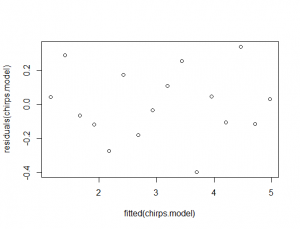This plots the fitted Frequency values (i.e. Frequency.fitted = 0.1271×Temperature-0.1140) as the x variable against the residual values (ε=Frequency-Frequency.fitted) as the y variable. If the residuals are behaving themselves (i.e. they are normal), this should look like a starry sky, with equal numbers of points above and below 0. If the residuals increase or decrease (i.e. it looks like you could stick a sloping line or a curve through them) with the fitted values, or are asymmetrically distributed, then your data break the assumptions of linear regression, and you should be careful in their interpretation.

You should also look at the normal quantile-quantile (QQ) plot of the residuals:

qqnorm( residuals( chirps.model ) )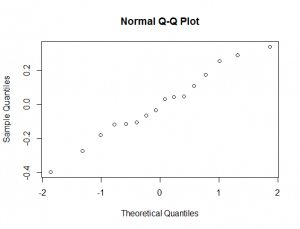The points on this graph should lie on a straight line. If they’re curved, again, your data break the assumptions of linear regression, and you should be careful in their interpretation. You can scan through these and other diagnostic plots using:

plot( chirps.model )

# Exercises

Fit linear models to the following data sets. Criticise the modelling: are the assumptions of the linear regression met?

1. Using the sycamore_seeds.csv file you have already made, model the data and add a suitable regression line to the graph. You saved the code for the graph, and the CSV file from before, didn’t you?
2. The file nadh_absorbance.csv contains data on the absorbance (A) at 340 nm of solutions containing increasing micromolar concentrations (C) of NADH. What is the Beer-Lambert molar extinction coefficient (ϵ) for NADH at 340 nm? How confident are you in your estimate? Is there anything about the data set that violates the linear regression assumptions? [Note that the epsilon here is the standard symbol for molar extinction coefficient and has nothing to do with the residuals]$A=\epsilon C l$
1. There is a relationship between the size of an island (or other defined area) and the number of species it contains. This relationship is modelled by the equation below, where S is the number of species, A is the area, and C and z are data-specific constants. Using logs, convert this equation into a linear form. Use R to transform the data below, and to estimate C and z. Criticise your model, and comment on the reliability of the results.$S=C A^z$
 Island Area of island / km2 Number of (non-bat) mammal species Jersey 116.3 9 Guernsey 63.5 5 Alderney 7.9 3 Sark 5.2 2 Herm 1.3 2

1. Sycamore seeds regression
sycamore.seeds<-read.csv( "H:/R/sycamore_seeds.csv" )
plot(
descent.speed ~ wing.length,
data = sycamore.seeds,
xlab = "Wing length / mm",
ylab = expression("Descent speed " / m*s^-1),
main = "Sycamore seeds with longer wings fall more slowly"
)
sycamore.seeds.model<-lm( descent.speed ~ wing.length, data=sycamore.seeds )
abline( sycamore.seeds.model )
summary( sycamore.seeds.model )
Call:
lm(formula = descent.speed ~ wing.length, data = sycamore.seeds)
Residuals:
Min        1Q   Median        3Q       Max
-0.073402 -0.034124 -0.005326  0.005395 0.105636
Coefficients:
Estimate Std. Error t value Pr(>|t|)
(Intercept)  2.388333   0.150479  15.872 9.56e-07 ***
wing.length -0.040120   0.004607  -8.709 5.28e-05 ***
---
Signif. codes:
0 '***' 0.001 '**' 0.01 '*' 0.05 '.' 0.1 ' ' 1
Residual standard error: 0.06416 on 7 degrees of freedom
Multiple R-squared:
R-squared:  0.9034
F-statistic: 75.85 on 1 and 7 DF,  p-value: 5.28e-05

Both the intercept and the slope are significantly different from zero. The slope is negative, around −0.04 m s−1 mm−1. The residuals don’t look too bad, but note that if you accept the model without thinking, you’ll predict that a wing of length −y-intercept/slopec. 60 mm (i.e. the x-intercept) would allow the seed to defy gravity forever. Beware extrapolation!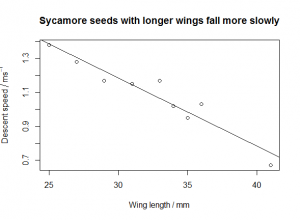nadh.absorbance<-read.csv( "H:/R/nadh_absorbance.csv" )
plot(
A340 ~ Conc.uM,
ylab = expression(A),
main = "Absorbance at 340 nm shows shows linear\nBeer-Lambert law for NADH"
)
summary( nadh.absorbance.model )
Call:
lm(formula = A340 ~ Conc.uM, data = nadh.absorbance)
Residuals:
Min         1Q     Median         3Q       Max
-0.0043482 -0.0020392 -0.0004086  0.0020603 0.0057544
Coefficients:
Estimate Std. Error t value Pr(>|t|)
(Intercept) 2.973e-03  8.969e-04   3.314  0.00203 **
Conc.uM     6.267e-03  3.812e-05 164.378  < 2e-16 ***
---
Signif. codes:
0 '***' 0.001 '**' 0.01 '*' 0.05 '.' 0.1 ' ' 1
Residual standard error: 0.002783 on 38 degrees of freedom
Multiple R-squared: 0.9986,    Adjusted R-squared:  0.9986
F-statistic: 2.702e+04 on 1 and 38 DF,  p-value: < 2.2e-16The slope is 6.267×10−3 µM, which means ϵ is 6.267×103 M. This is very significantly different from zero; however, so too is the intercept, which – theoretically – should be zero. You’ll also note that the Q-Q plot:

qqnorm( residuals( nadh.absorbance.model ) )Is very clearly not a straight line, indicating that the variance in the residuals is not a constant.

1. Species-area requires a log/log transformation$\log{S} = \log{C}+z\log{A}$
logS<-log(c(     9,    5,   3,   2,   2 ))
logA<-log(c( 116.3, 63.5, 7.9, 5.2, 1.3 ))
species.area<-data.frame(logS=logS,logA=logA)
plot(
logS ~ logA,
data = species.area,
xlab = expression("ln( Area of island"/ km^2 *" )"),
ylab = "ln( Number of species )",
main = "Species supported by islands of different areas"
)
species.area.model<-lm( logS ~ logA, data=species.area )
abline( species.area.model )
summary( species.area.model )
Call:
lm(formula = logS ~ logA, data = species.area)

Residuals:
1        2        3        4        5
0.22137 -0.16716  0.00828 -0.25948  0.19699

Coefficients:

Estimate Std. Error t value Pr(>|t|)
(Intercept) 0.40977    0.20443   2.004   0.139
logA        0.32927    0.06674   4.934   0.016 *
---
Signif. codes:
0 '***' 0.001 '**' 0.01 '*' 0.05 '.' 0.1 ' ' 1

Residual standard error: 0.2471 on 3 degrees of freedom
Multiple R-squared: 0.8903,    Adjusted R-squared:  0.8537
F-statistic: 24.34 on 1 and 3 DF,  p-value: 0.01597

log C is the (Intercept), 0.4098 (so C itself is e0.4098 = 1.5), and z is the slope associated with logA, 0.329 km−2. The residual plots are a little difficult to interpret as the sample size is small; and you’ll note the large error in the estimate of log C, which is not significantly different from 0 (i.e. C may well be 1). I wouldn’t want to bet much money on the estimates of C or of z here.

This site uses Akismet to reduce spam. Learn how your comment data is processed.